## Wind Power

Wind power is one type of renewable energy. The wind can blow the blades of a wind turbine and then drive the main shaft which connects with the rotor of a generator. Depending on the wind speed, the angular speed of the main shaft changes and generates a corresponding electrical current. In this simple example, we practice two examples of wind power and using some sensors to measure the power loss in each phase.

### Wind turbine

In the first example, we integrate a wind turbine into the main grid of 3-phase AC voltage source as below. We need a wind turbine, a 3-phase AC voltage source, a generator which transforms the mechanical power to the electrical power, and an AC/AC converter which transforms the voltage or frequency level. For getting the power loss, we also use a mechanical power sensor and an AC circuit power sensor.To put a wind turbine, left-click Components/Library/GreenEnergy/Wind/WindTurbine (step 1) and release the mouse. The wind turbine follows the cursor and left-click to put it on the workscreen. Right-click on the wind turbine (step 2) to change its parameters. In the pop-window, we can see the parameters as below (step 3):

• A = 4500 m� (turbine swept area)
• Friction = 250 Nm/Rad/s (friction of the rotor)
• Inertia = 6MEG Kgm� (inertia of the rotor)
• Initial Rotor Speed = 0 Rad/s (initial speed of the turbine)
• Rho = 1.2 (air density)

Click OK to save the setting (step 4).Then give a generator to transform the mechanical power to the electrical power. Left-click Components/Library/ElectricalMachines/System/Generator (step 1) and release the mouse. The generator follows the cursor and left-click to put it in the right side of the wind turbine (step 2). Notice to keep a bit space between the wind turbine and the generator in order to insert a power sensor later. Right-click the generator (step 2) to modify its parameters if needed. In the pop-window, we can see the parameters as below (step 3):

• Efficiency[from 0 to 1] = 0.95 (efficiency of the generator)
• Minimum Angular Speed = 1 Rad/s (if the angular speed is below this minimum angular speed value, the power consumption changes from constant power into constant braking torque)
• Power factor[from 0 to 1] = 1 (the power factor or cos(phi) between the voltage and current vector because the generator has only sinusoidal outputs)

Click OK to save the setting (step 4).To let the AC/AC converter adjust its parameters according to the mechanical power, we add a power optimizer which measures the angular speed of the main shaft and then calculate the power value for the AC/AC converter.

Left-click Components/Library/GreenEnergy/Wind/OptimumPower (step 1) and release the mouse. The power optimizer follows the cursor and left-click to put it below the generator (step 2). Right-click the power optimizer to change its parameter if needed. The parameter we have here is the constant value K (step 3). Click �Help Lib� to see how K works for calculating the power value (step 4). In the pop-window, we can see that the torque value from the main shaft T= K * &omega * &omega, so that the power = torque * angular speed = T * &omega. Click OK to save the setting if needed (step 5).

Connect the power optimizer and the AC/AC converter as the following setting (step 6). Its input is to sense the angular speed of the main shaft; its output is to calculate the power value and then send this value to the AC/AC converter.To connect the AC/AC converter with the main grid, we put a 3-phase voltage source in the right side of the converter. Left-click Components/Library/Source/Circuit/ThreePhase/VoltageSourceRMS (step 1) and release the mouse. The voltage source follows the cursor and left-click to put it in the left side of the AC/AC converter (step 2). Right-click on the voltage source to change its parameters. In the pop-window, we can see its parameters as below (step 3):

• Frequency = 50 Hz
• Vrms = 230 (RMS voltage)
• Phase [from 0 to 360] = 0 (phase in degrees)
• Select �Flip Vertical� (step 4) in the rotation setting

Click OK to save the setting (step 5).Left-click Components/Library/Sensor/Rotational/PowerTorque (step 1) and release the mouse. Left-click on the workscreen to connect the power torque sensor with the generator (step 2).

Left-click Components/Library/Sensor/Electrical/PowerAC3Phase (step 3) and release the mouse. Left-click on the workscreen to connect the AC power sensor with the AC/AC converter (step 4).Connect the wind turbine and the power torque sensor (step 1). Connect the voltage source and the AC power sensor (step 2). Currently we have not set an electric ground so that right-click on the neutral input of the voltage source to add a ground label (step 3). In the pop-window, enter label = Ground (step 4) and click OK to save the setting (step 5).Make sure there is a ground label in the neutral input of the voltage source (step 1).

Add an UI2RST sensor to read the RST signals of the voltage�s and the current�s vectors. Left-click Components/Library/sensor/Electrical/ui2rst (step 2) and release the mouse. Left-click on the workscreen to put the UI2RST sensor below the AC power sensor (step 3).

Then define the wind speed for the wind turbine. Right-click the wind speed input (step 4) and in the pop-window, enter label = 8 (which stands for the wind speed = 8m/s) (step 5). Click OK to save the setting (step 6).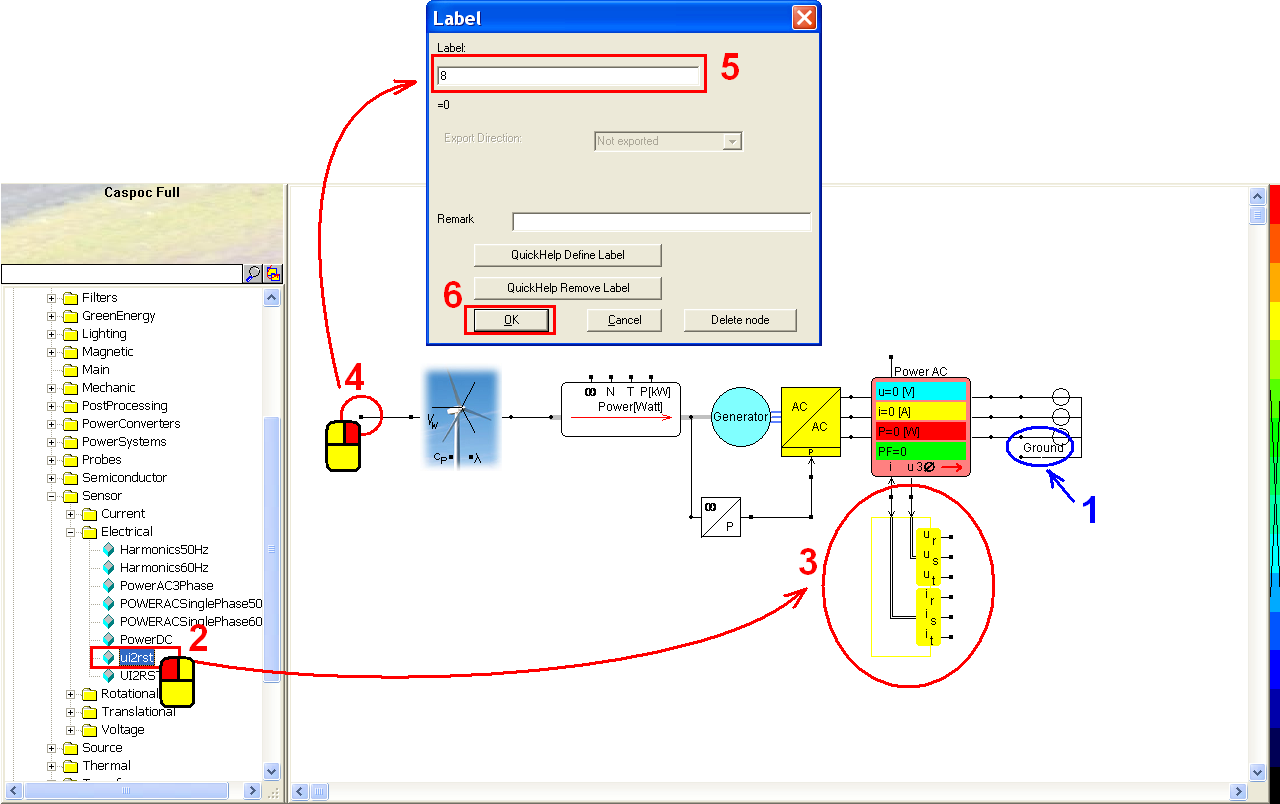Then give a scope to read the power values from these power sensors. Click the scope iconin the experience bar (step 1) and release the mouse. Left-click to put the scope above the AC power sensor. Left-click the right-bottom corner of the scope and hold down the mouse to enlarge this scope (step 2).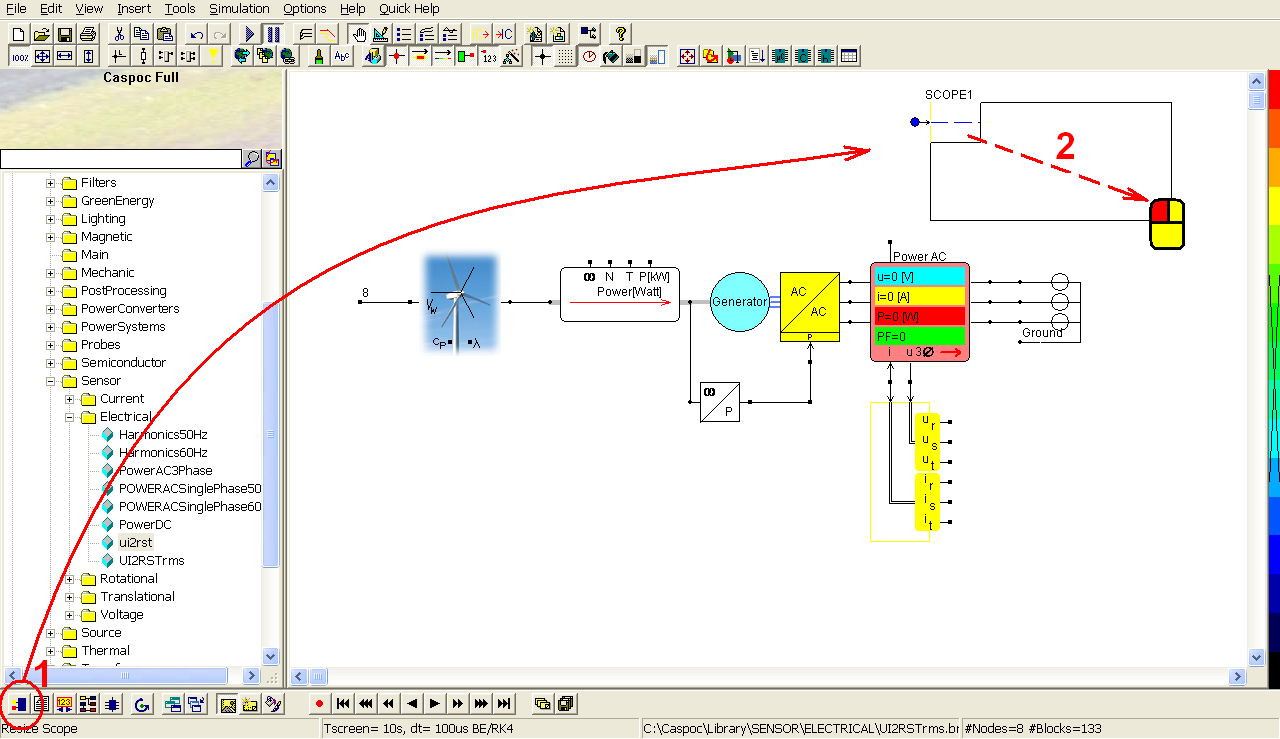Connect the fourth output (P[kW]) of the power torque sensor to the first blue input trace of the first scope (step 1). Also the power output of the AC power sensor to the second red input trace. Right-click the first scope (step 2) and in the pop-window, select Trace/Trace1 (step 3). In the pop-window of the Trace1, set the Scale in the right side (step 4) and click OK to save the setting (step 5).

Click the scope iconin the experience bar (step 6) and release the mouse. Left-click to put the second scope in right side of the UI2RST sensor. Make sure that the first blue input trace is connected with the first output Ur of the UI2RST sensor. Left-click the right-bottom corner of the scope and hold down the mouse to enlarge this scope (step 7). Then the 6 input trace of the second scope shall be well connected to Ur, Us, Ut, Ir, Is and It. If not, adjust the position of second scope to make sure that all the connections are well done.Click the short-cut of simulation parameter(step 1). In the pop-window, select Euler for the Numerical Integration Method (step 2), Tscreen = 100, dt = 100u (step 3) and then click OK to save the setting (step 4).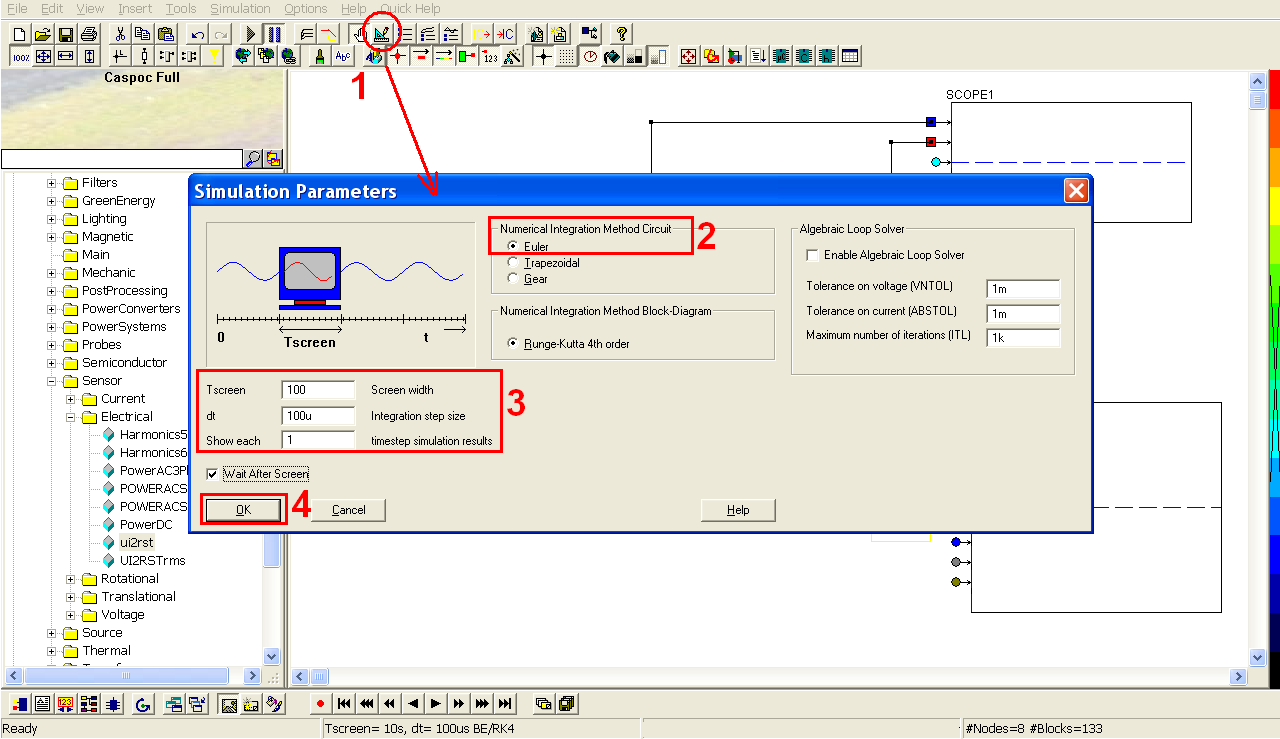Click the short-cut of start simulation(step 1). Right-click on the first scope (step 2) to see the details of the simulation result. Click the shortcut of listbox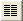(step 3) to enable the numeric display of simulation result. Click Right or Left key to find the precise values according to the time (step 4) and then we can find that the relation between the mechanical power and the AC electrical power depends on the efficiency of the generator (step 6): 677.867 kW * 0.95 = 643.97365 kW ~ 643.656kW.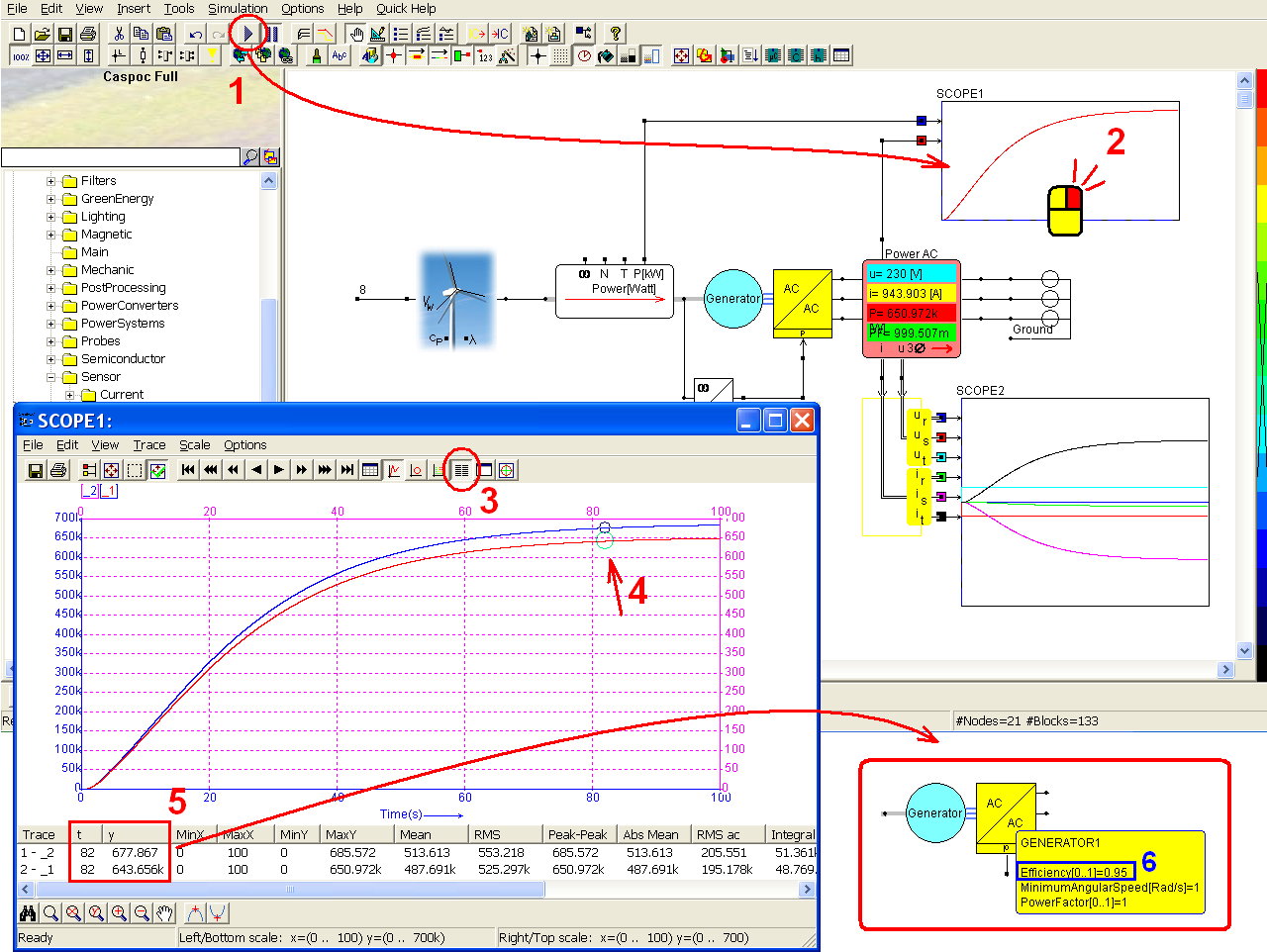### 3-phase rectifier for a wind turbine

In the second example, we remove the main grid but add a RL circuit to be an electrical load for the wind power. As well as the previous example, we use a generator namely AC motor to convert the mechanical power to electrical power. Then convert the alternating current to direct current by using a rectifier, that is, AC/DC converter. We also add power sensors to measure the power loss in each phase as the following configuration.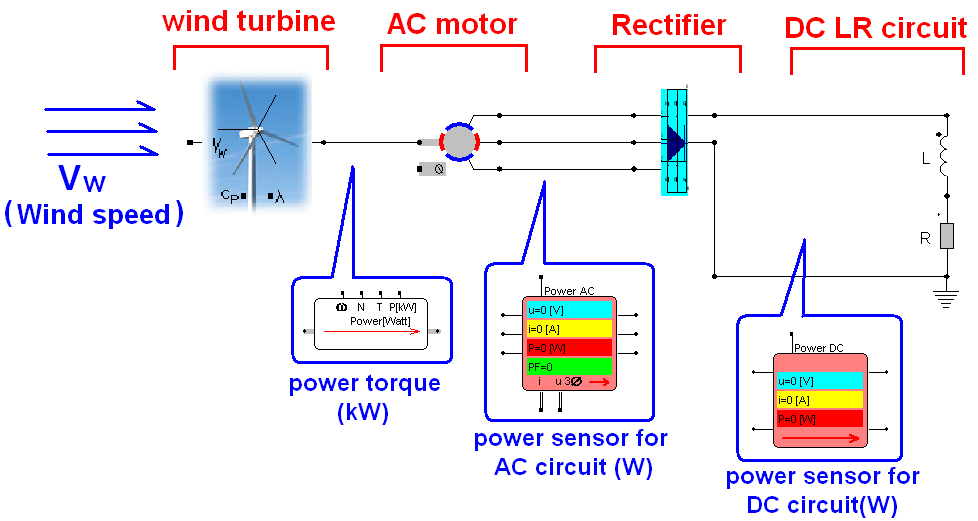Continue the second example by the previous example. Left-click and draw the mouse to select all the components except the wind turbine and the power torque sensor (step 1). Click the Delete key to remove the components we selected (step 2).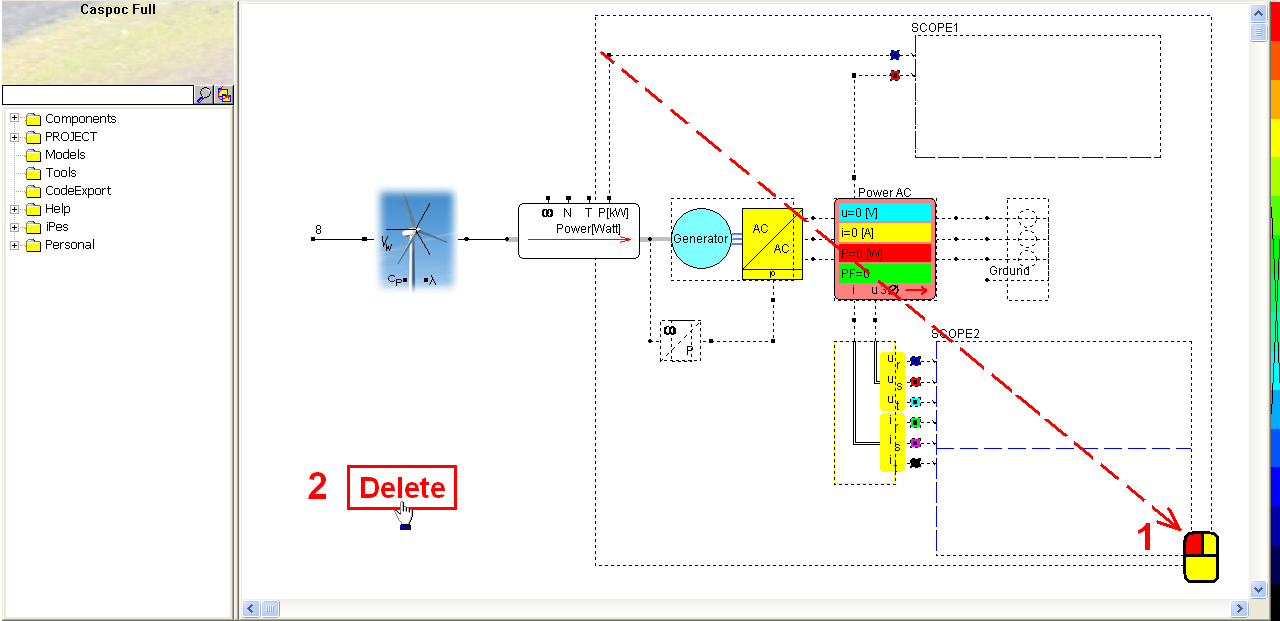Right-click the wind turbine (step 1). In the pop-window, modify the parameters of the wind turbine as below (step 2):

• A = 6.6 m� (turbine swept area)
• Friction = 1m Nm/Rad/s (friction of the rotor)
• Inertia = 1 Kgm� (inertia of the rotor)
• Initial Rotor Speed = 0 Rad/s (initial speed of the turbine)
• Rho = 1.2 (air density)

Click OK to save the setting (step 3).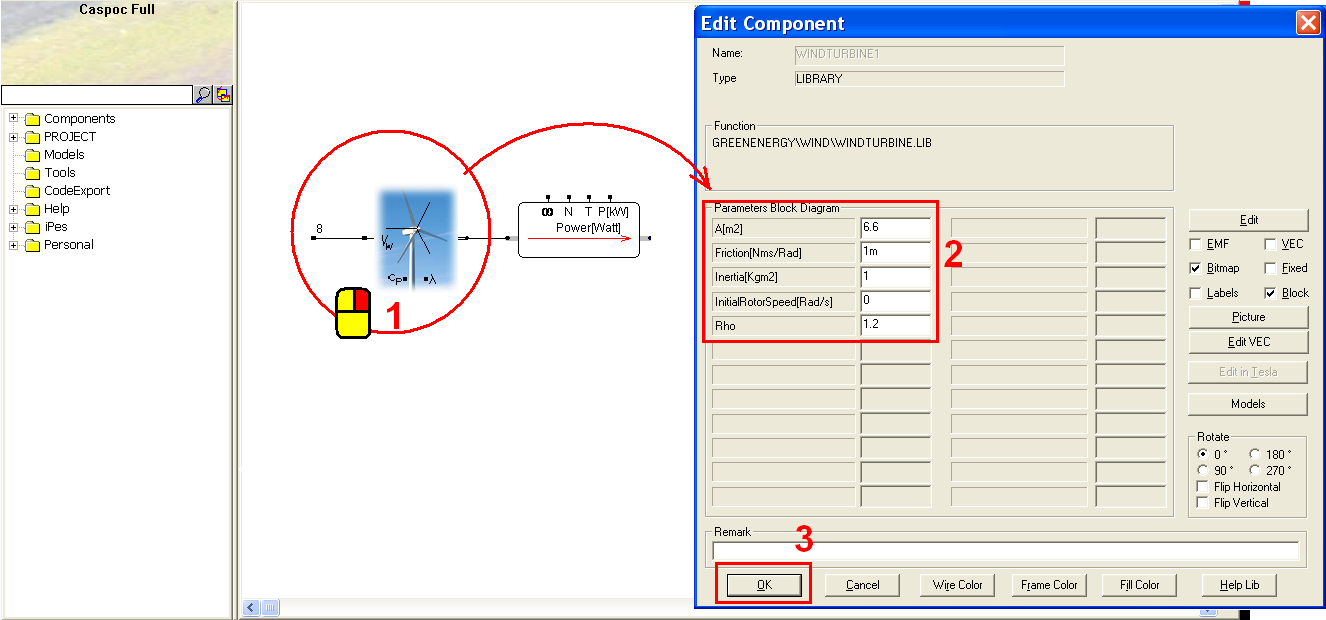Left-click Components/Library/ElectricalMachines/PMSM/PMSM (step 1) and release the mouse. The PMSM follows the cursor and left-click on the workscreen to put it in the right side of the power torque sensor (step 2). Right-click the PMSM to modify its parameters. In the pop-window, we can see the parameters as below:

• Friction = 1m Nm/Rad/s (friction of the bearing)
• JR = 100m Kgm� (rotor inertia)
• Ke = 1 [Vpeak/(Rad/s) = Nm/A] (torque / back EMF constant)
• LS = 15mH (winding inductance per phase)
• RS = 10m ohm (winding resistance per phase)
• Rm = 1000 (the resistance in parallel with the magnetizing inductance for modeling the eddy current and hysteresis losses in the electrical machine)
• Polepair = 12 (number of pole pairs) (step 3)
• Select �Flip Vertical� (step 4) in the rotation setting

Click OK to save the setting (step 5).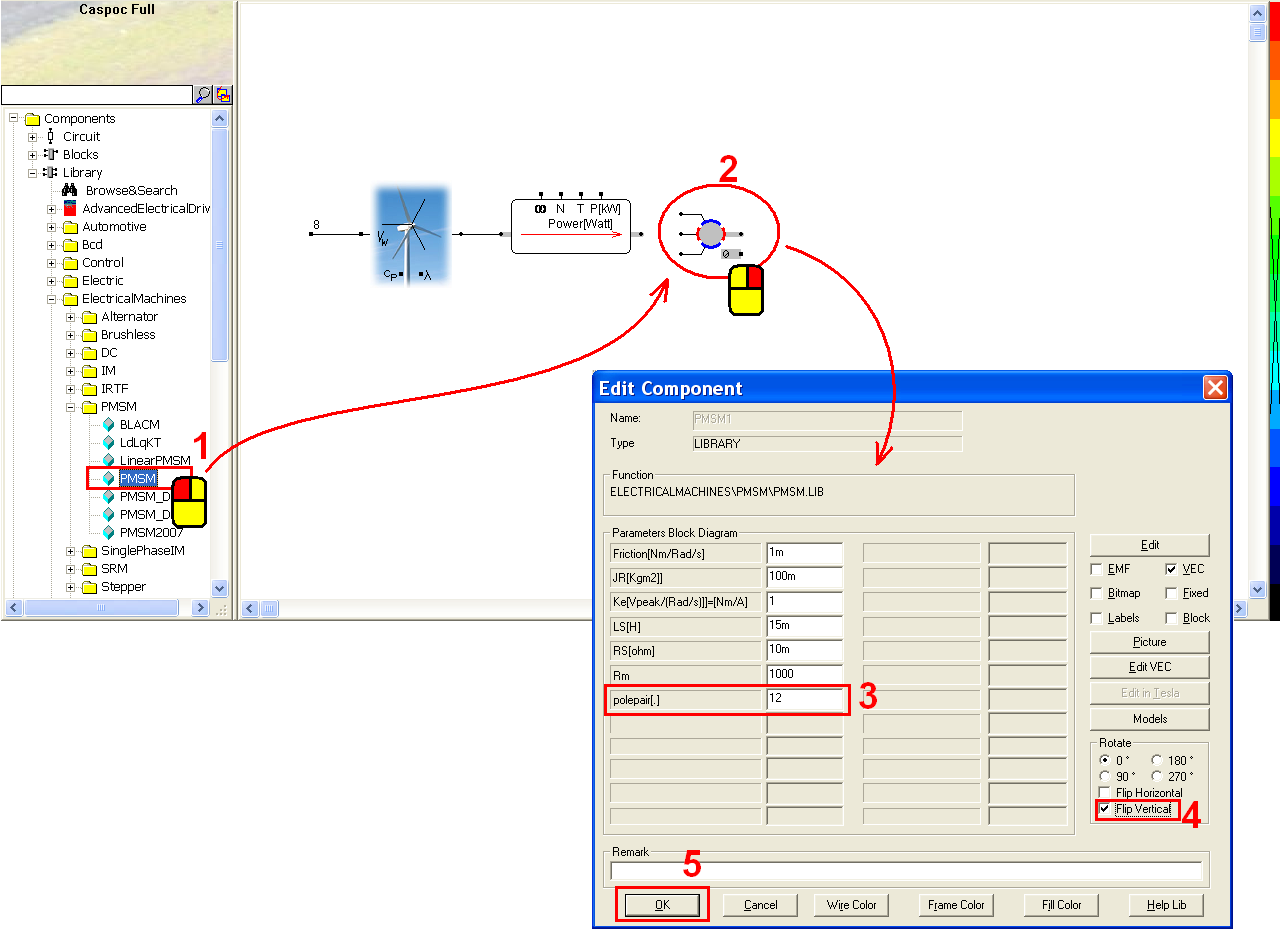Connect the PMSM and the power torque sensor (step 1). Left-click Components/Library/Sensor/Electrical/PowerAC3Phase (step 2) and release the mouse. The AC power sensor follows the cursor and left-click to put it on the workscreen and connect with the PMSM (step 3).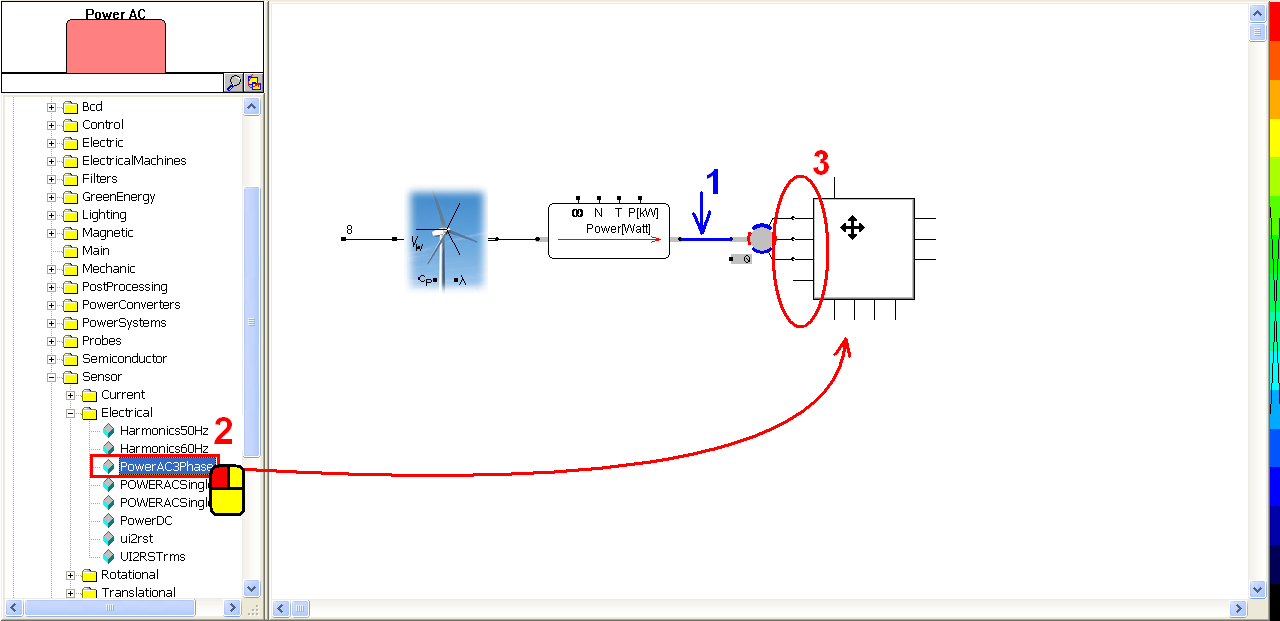Now use a rectifier to convert the alternating current. Left-click Components/Library/PowerConverters/Rectifier3Phase/b6 (step 1) and release the mouse. The rectifier follows the cursor and left-click to put it in the right side of the AC power sensor (step 2). Right-click the rectifier to see its parameters as below (step 3):

• BV = 100k Volt (break down voltage per diode)
• Roff = 1e6 ohm (blocking resistance of the diode)
• Ron = 1m ohm (on resistance of the diode)
• Von = 0.6 volt (conduction on state voltage)

Click �Help Lib� to see the explanation of these parameters (step 4). If any parameter is modified, click OK to save the setting (step 5).Before giving a RL circuit, we place a DC power sensor in the right side of the rectifier. Left-click Components/Library/Sensor/Electrical/PowerDC (step 1) and release the mouse. Left-click on the workscreen to put the DC power sensor in the right side of the rectifier (step 2). Make sure the upper DC input of the DC power sensor is well connected with the DC output of the rectifier. Connect the left ground of the DC power sensor and the ground of the rectifier (step 3).Left-click Components/Circuit/RLC/L (step 1) and release the mouse. The inductor follows the cursor and right-click to change its direction if needed (step 2). Until the anode of the inductor (indicated by the little dot) is upward, left-click to put it in the right side of the DC power sensor. Right-click the inductor to modify its value (step 3). In the pop-window, enter value = 100m (step 4) and click OK to save the setting (step 5).Left-click Components/Circuit/RLC/R (step 1) and release the mouse. The resistor follows the cursor and right-click to change its direction if needed (step 2). Until the anode of the resistor (indicated by the little dot) is upward, left-click to put it below the inductor. Right-click on the resistor to modify its value (step 3). In the pop-window, enter value = 200 (step 4) and click OK to save the setting (step 5).Connect the RL circuit with the DC power sensor with the following configuration (step 1). Currently we have not set an electrical ground. Right-click the cathode of the RL circuit to add a ground label (step 2). In the pop-window, enter label = Ground (step 3) and click OK to save the setting (step 4).Left-click the power value output of the power torque sensor (step 1) to add a label. In the pop-window, enter label = P_m (step 2) and click OK to save the setting (step 3). Left-click the power value output of the AC power sensor (step 4) to add a label. In the pop-window, enter label = P_ac (step 5) and click OK to save the setting (step 6). Left-click the power value output of the DC power sensor (step 7) to add a label. In the pop-window, enter label = P_dc (step 8) and click OK to save the setting (step 9).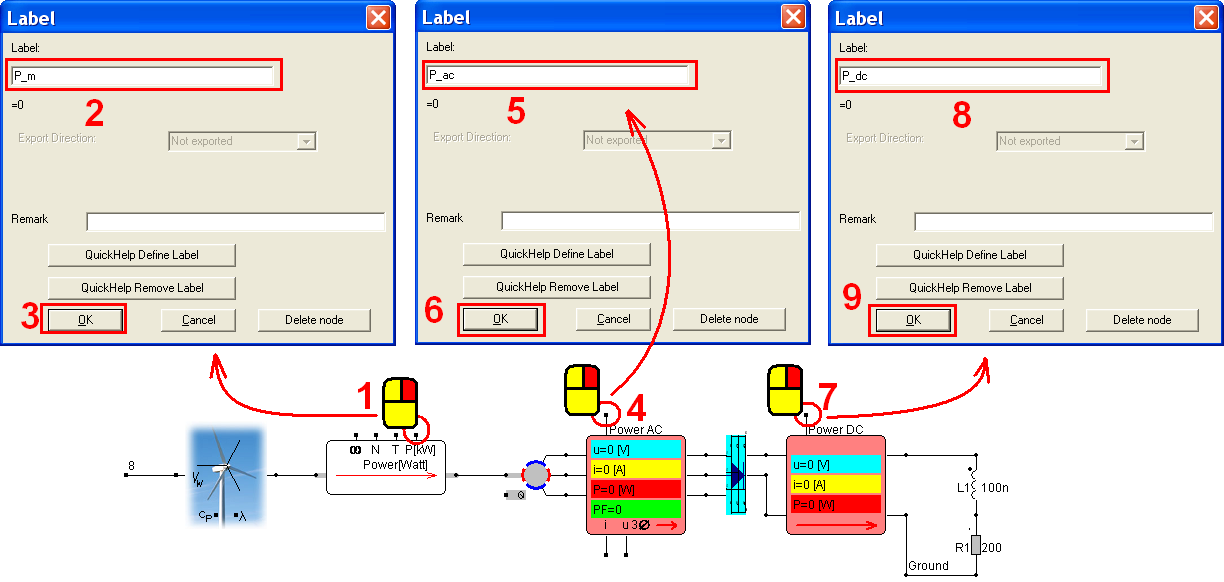Now take some scopes to record the simulation result. Click the scope iconin the experience bar (step 1) to put a scope below the wind turbine. Left-click the right-bottom corner of the scope and hold down the mouse to enlarge this scope (step 2). Likewise, click the scope iconin the experience bar (step 3) to put a scope below the AC power sensor. Left-click the right-bottom corner of the scope and hold down the mouse to enlarge this scope (step 4).Connect the T phase of the AC motor with the first blue input trace of the second scope (step 1) so that the voltage value of T phase will be shown in this scope.

In the first scope, left-click the first blue input trace and draw it leftward a bit to extend its length (step 2). Repeat the same steps with the second red and the third azure input traces. Right-click the first input trace (step 3) to add a label. In the pop-window, enter label = P_m (step 4) and click OK to save the setting (step 5). Right-click the second input trace (step 6) to add a label. In the pop-window, enter label = P_ac (step 7) and click OK to save the setting (step 8). Right-click the third input trace (step 9) to add a label. In the pop-window, enter label = P_dc (step 10) and click OK to save the setting (step 11).Click the scope iconin the experience bar (step 1) to put a scope in the right side of the inductor (step 2). Connect the first input trace of the third scope with the anode of the inductor (step 3). Click the scope iconin the experience bar (step 4) to put a scope in the right side of the resistor (step 5). Right-click its first input trace (step 6). In the pop-window, enter Trace=Current through: R1 (step 7) and click OK to save the setting (step 8).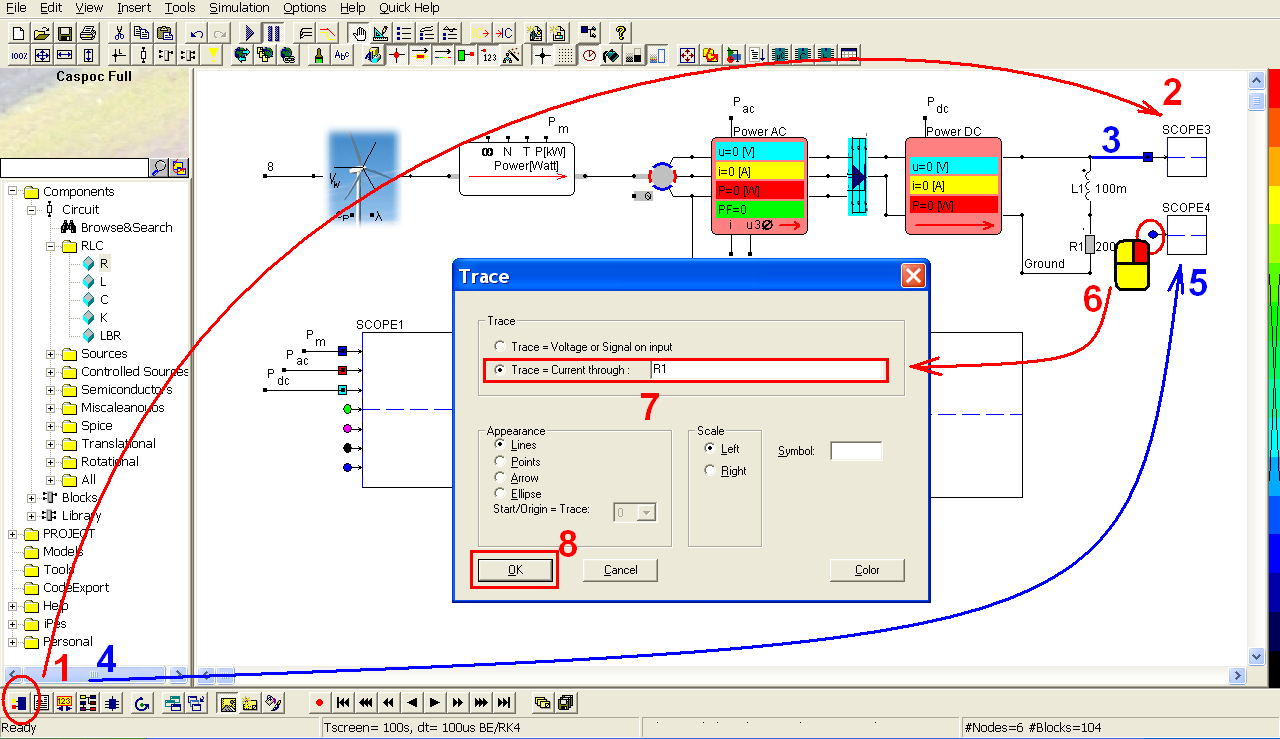click the short-cut of simulation parameter(step 1). In the pop-window, select Euler for the Numerical Integration Method (step 2), Tscreen = 10, dt = 100u (step 3) and then click OK to save the setting (step 4).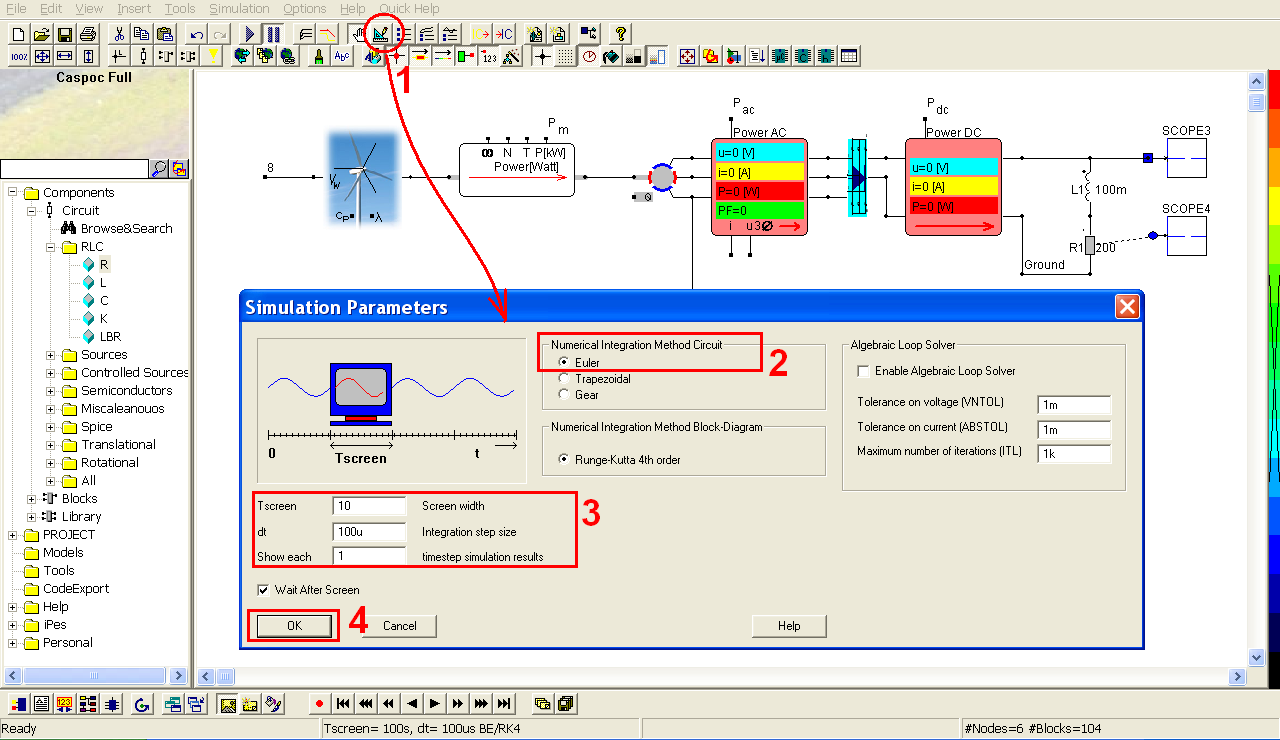Click the short-cut of start simulation(step 1). Right-click on the first scope (step 2) to see the details of the simulation result. Click the shortcut of listbox(step 3) to enable the numeric display of simulation result. Click Right or Left key to find the precise values according to the time (step 4). Then we can see clearly the power loss after the AC motor and the rectifier (step 5).